# The Convolution Theorem with Application Examples¶

The convolution theorem is a fundamental property of the Fourier transform. It is often stated like

"Convolution in time domain equals multiplication in frequency domain"

or vice versa

"Multiplication in time equals convolution in the frequency domain"

In this notebook we will illustrate what that means by pictorial examples. First, let us state the first version of the theorem mathematically. Let $x(t)$ and $y(t)$ be two arbitrary signals. Then, we have

$$\mathcal{F}\{x(t)*y(t)\}(f)=\mathcal{F}\{x(t)\}(f)\cdot\mathcal{F}\{y(t)\}(f),$$

where $\mathcal{F}\{x(t)\}(f)$ denotes the Fourier transform of $x(t)$, evaluated at the frequency $f$. In other words, we can say:

"The spectrum of the convolution two signals equals the multiplication of the spectra of both signals"

Let us first recap convolution (a more detailed description is given in another article on convolution): Given two signals $x(t)$ and $y(t)$, their convolution is defined by $$z(t)=x(t)*y(t)=\int_{-\infty}^{\infty}x(\tau)y(t-\tau)d\tau.$$

Literally, we take one signal, mirror it in time and shift it in time domain. Then we multiply this signal with the other signal and calculate the integral of the overlapping part. Let us now calculate the convolution of two arbitrary signals and look at the result in time and frequency domain. Here, we consider a continuous-time signal, which we discretize in time domain with sampling frequency $F_s=100Hz$.

Fs = 100    # sampling frequency
T = 10      # time duration we want to look at
t = np.arange(-T, T, 1/Fs)  # the corresponding time samples

# define our two functions
x = lambda t: np.exp(-abs(t)) * (t>=0)
y = lambda t: np.sinc(t)**2

# the resulting time range, when convolving both signals
t_conv = np.arange(-2*T, 2*T, 1/Fs)[:-1]

plt.subplot(121)
plt.plot(t, x(t), label='$x(t)$')
plt.plot(t, y(t), label='$y(t)$')
z = np.convolve(x(t), y(t))/Fs
plt.plot(t_conv, z, label='$z(t)$')

plt.subplot(122)
# function to calculate the spectrum of the input signal
spec = lambda x: abs(np.fft.fftshift(np.fft.fft(x, 4*len(t))))/Fs
X = spec(x(t))
Y = spec(y(t))
Z = spec(z)
f = np.linspace(-Fs/2, Fs/2, len(X))
plt.plot(f, X, label='$|X(f)|$')
plt.plot(f, Y, label='$|Y(f)|$')
plt.plot(f, Z, label='$|Z(f)|=|X(f)\\cdot Y(f)|$')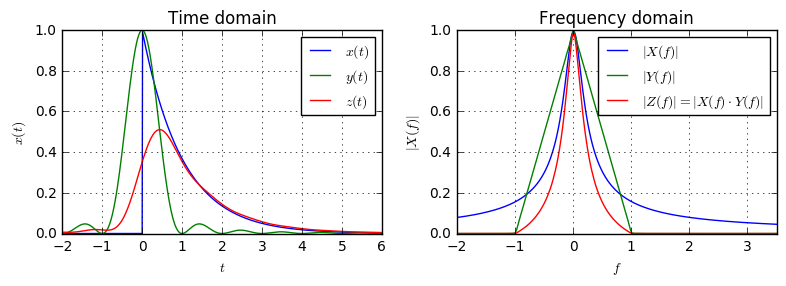In the above example, we have convolved an exponential impulse $x(t)$ with a sinc-squared function $y(t)$. The result of the convolution $z(t)$ is shown in the red curve. On the left-hand side, we can look at the time domain. In the diagram on the right side, the spectrum of all signals is shown. We see that the spectrum of the exponential impulse is very wide in frequency domain (this is clear, since we have the abrupt jump at $t=0$ in the time domain). On the other side, the sinc-squared function corresponds to a triangle in the frequency domain with $|Y(f)|=0, |f|\geq1$. Eventually, the red curve is the product of the green and blue curves: For $f=0$, it starts at $|Z(f)|_{f=0}=1$ and decays with increasing $f$. At $f=1$, where the green triangle has decayed to zero, also the red curve decayed to zero.

Let us now look at another example: We convolve a Gaussian bell function with an ideal lowpass of different cutoff frequencies. Since the ideal lowpass has an infinite time domain response which decays rather slow, we add a Hanning window to the function such that the resulting spectra will look more smooth.

gauss = lambda t: np.exp(-0.2*t*t) / 4 # The Gaussian Bell function
def lowpass(fc): # return impulse response of the ideal lowpass with cutoff fc
return lambda t: 2*fc * np.sinc(2*fc*t)

def showConvolution(x, y):
Fs = 100                    # signal sampling frequency
T = 100                     # time duration for the impulse response to decay
t = np.arange(-T, T, 1/Fs)  # the time samples
# the time samples of the signal after convolution
t_convolved = np.arange(-2*T, 2*T, 1/Fs)[:-1]

# Get the samples of the signals. Multiply with Hanning window
# to mitigate ringing/spectral leakage in the frequency domain
x_samples = x(t) * np.hanning(len(t))
y_samples = y(t) * np.hanning(len(t))
z_samples = np.convolve(x_samples, y_samples) / Fs

spec = lambda x: np.fft.fftshift(np.fft.fft(x, 16*len(t))) / Fs
X_samples = spec(x_samples)
Y_samples = spec(y_samples)
Z_samples = spec(z_samples)
f = np.linspace(-Fs/2, Fs/2, len(X_samples), endpoint=False)

plt.subplot(121)
plt.plot(t, x_samples, label='$x(t)$')
plt.plot(t, y_samples, label='$y(t)$')
plt.plot(t_convolved, z_samples, label='$z(t)$')

plt.subplot(122)
plt.plot(f, abs(X_samples), label='$|X(f)|$')
plt.plot(f, abs(Y_samples), label='$|Y(f)|$')
plt.plot(f, abs(Z_samples), label='$|Z(f)|$')Run code interactively  Hide



As we see, the ideal lowpass is a rectangular function in the frequency domain, whereas the Gaussian remains to be a Gaussian in frequency. As long as the cutoff frequency of the lowpass is large enough, such that the blue curve in frequency domain is within the green rect, the convolution of the ideal lowpass with the Gaussian remains to be a Gaussian. This is clear, since we see in the frequency domain that the spectrum of the original signal is not changed (actually, it is marginally changed, since the Gaussian is infinitely wide in frequency). However, as soon as the green rect cuts a significant portion of the blue spectrum, the convolution of both signals is not a Gaussian anymore, and the blue and red curve deviate in time domain.

## Computing convolution via the frequency domain¶

The convolution theorem can be beneficially used to compute the convolution of two signals. Rephrasing the convolution theorem, we get

$$x(t)*y(t)=\mathcal{F}^{-1}\{\mathcal{F}\{x(t)\}\cdot\mathcal{F}\{y(t)\}\},$$

i.e. to calculate the convolution of two signals $x(t)$ and $y(t)$, we can do three steps:

1. Calculate the spectrum $X(f)=\mathcal{F}\{x(t)\}$ and $Y(f)=\mathcal{F}\{y(t)\}$.
2. Calculate the elementwise product $Z(f)=X(f)\cdot Y(f)$
3. Perform inverse Fourier transform to get back to the time domain $z(t)=\mathcal{F}^{-1}\{Z(f)\}$

Even though this sounds more complicated, it can actually save a lot of computation resources. Since the calculation in the time-domain equals a double summation (or solving an integral for each time-domain output), the runtime in time-domain can actually be quadratic. In frequency domain only a simple multiplication needs to be performed, leading to linear complexity. However, still the transformation to frequency and time via Fourier transform needs to be done. But, using the Fast-Fourier-Transform algorithm, this can also be done with small complexity.

Lets directly check, if the technique works: We take two sequences of length $N=128$, one being the exponential impulse, one being a sine wave and calculate the conovolution once in time domain and once in the frequency domain:

N = 128
n = np.arange(N)

x_samples = np.exp(-0.1*n)
y_samples = np.sin(2*np.pi*n/32)
z_samples = np.convolve(x_samples, y_samples)
# Application of the convolution theorem:
z2_samples = np.fft.ifft(np.fft.fft(x_samples) * np.fft.fft(y_samples)).real

plt.subplot(121)
plt.plot(x_samples, label='$x(t)$')
plt.plot(y_samples, label='$y(t)$')
plt.plot(z_samples, label='$z(t)$')

plt.subplot(122)
plt.plot(x_samples, label='$x(t)$')
plt.plot(y_samples, label='$y(t)$')
plt.plot(z2_samples, label='$z(t)$');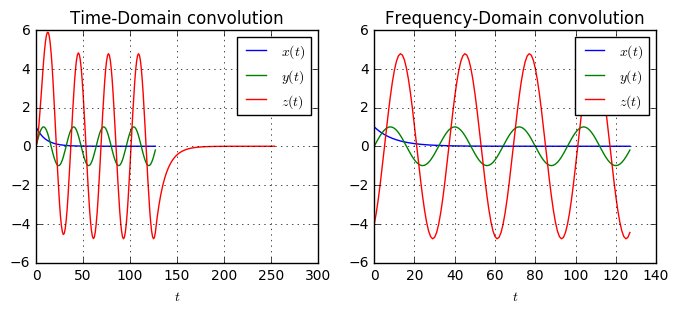As we see, the red curves on the left and right figures look very different. What is going on here? Is the convolution theorem wrong? No, it's not wrong, but the problem is the application of the discrete Fourier transform here. As shown in an article about the DFT properties, the DFT considers its input to be one period of a periodic signal. Hence, when applying convolution using the DFT, it actually performs a circular convolution rather than a linear/conventional convolution. So, for the DFT case, the convolution theorem is actually true for the circular convolution.

What can we do, to still use the DFT to perform the convolution? We can perform zero-padding of the signals before convolution. In time domain, this does not change the convolution result, because adding zeros to the signal wont change it. However, by knowing that the DFT performs circular convolution, we need to provide enough space in time domain, such that the convolution does not wrap around (or wraps only zeros around).

How much space do we need to provide? We know, that the length of the convolution of two sequences of length $L_1$ and $L_2$ samples equals $L=L_1+L_2-1$ samples. Hence, we need to zero-pad our signals at least to this length. Let's try this:

N = 128
n = np.arange(N)
Z = np.zeros(N)

x_samples = np.hstack([np.exp(-0.1*n), Z])
y_samples = np.hstack([np.sin(2*np.pi*n/32), Z])
z_samples = np.convolve(x_samples, y_samples)
z2_samples = np.fft.ifft(np.fft.fft(x_samples) * np.fft.fft(y_samples)).real

plt.figure(figsize=(8,3))
plt.subplot(121)
plt.plot(x_samples, label='$x(t)$')
plt.plot(y_samples, label='$y(t)$')
plt.plot(z_samples, label='$z(t)$')

plt.subplot(122)
plt.plot(x_samples, label='$x(t)$')
plt.plot(y_samples, label='$y(t)$')
plt.plot(z2_samples, label='$z(t)$');
plt.xlim((0,2*N));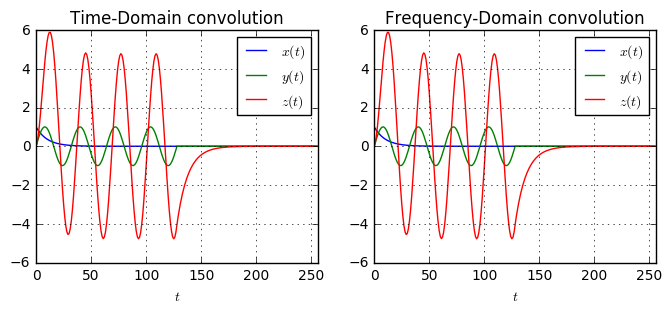Now, the convolution in time and frequency domain yield the same result. Performing the convolution in the frequency domain is called Fast convolution and there exist more elaborate algorithms on how to perform it numerically efficient.

## More examples¶

In the following we will show some examples where the knowledge of the convolution theorem can be helpful

Calculate $z(t)=x(t)*y(t)$ with $x(t)=\cos(2\pi f t)$ and $y(t)=\cos(2\pi 2f t)$ for all times.

Let's evaluate numerically. Since we are concerned with periodic signals and the signals should be repeated over all times, this is the perfect application for the circular convolution. Let us set $f=2Hz$. and do the calculation:

f = 2
x = np.cos(2*np.pi*f*t)
y = np.cos(2*np.pi*2*f*t)
z = np.fft.ifft(np.fft.fft(x)*np.fft.fft(y)).real

plt.subplot(121)
plt.plot(t, x, label='$x(t)$')
plt.plot(t, y, label='$y(t)$')
plt.plot(t, z, label='$z(t)$')

plt.subplot(122)
plt.plot(f, abs(np.fft.fftshift(np.fft.fft(x)))/(T*Fs), '-x')
plt.plot(f, abs(np.fft.fftshift(np.fft.fft(y)))/(T*Fs), '-x')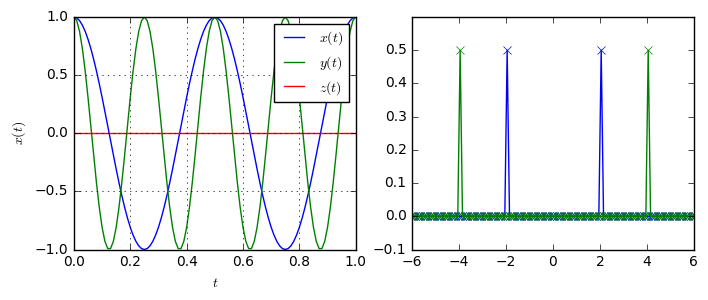As we can see, the convolution of the two sines equals constantly 0, i.e. $x(t)*y(t)=0$. Looking at the frequency domain of both signals, this is totally clear: Since we know the correspondence

$$\mathcal{F}\{\cos(2\pi f_0t)\}=\frac{1}{2}(\delta(f-f_0)+\delta(f+f_0)),$$

we can immediately see that the convolution of two cosines with different frequency equals zero, since the signals do not overlap in the frequency domain.

What is the convolution of $x(t)*y(t)$ for $x(t)=sinc(t)$ and $y(t)=2sinc(2t)$?

Let's calculate it:

x = np.sinc(t) * np.hanning(len(t))
y = 2*np.sinc(2*t) * np.hanning(len(t))
z = np.convolve(x, y) / Fs

plt.subplot(121)
plt.plot(t, x, lw=3, label='$x(t)$')
plt.plot(t, y, label='$y(t)$')
plt.plot(t_conv, z, label='$z(t)$')

plt.subplot(122)
plt.plot(f, abs(np.fft.fftshift(np.fft.fft(x)))/(Fs), lw=3, label='$X(f)$')
plt.plot(f, abs(np.fft.fftshift(np.fft.fft(y)))/(Fs), label='$Y(f)$')
plt.plot(f, abs(np.fft.fftshift(np.fft.fft(z)))[::2]/Fs, label='$Z(f)$')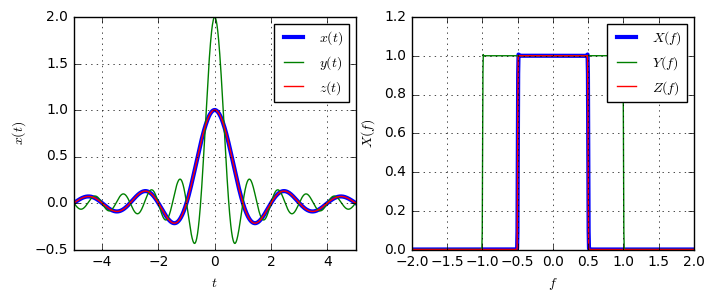As shown, we have $sinc(t)*sinc(2t)=sinc(t)$. Despite being quite difficult to calculate in the time domain via the convolution integral, looking at the frequency domain of both signals the answer becomes straight-forward: The multiplication of two rectangles with different widths equals the rectangle with the smaller width.

## Conclusion¶

The convolution theorem connects the time- and frequency domains of the convolution. Convolving in one domain corresponds to elementwise multiplication in the other domain.

The convolution theorem can be used to perform convolution via multiplication in the time domain.

The convolution theorem can be used benefically for calculation of some convolutions that would be difficult to solve with the convolution integral.Do you have questions or comments? Let's dicuss below!

### Related posts

DSPIllustrations.com is a participant in the Amazon Services LLC Associates Program, an affiliate advertising program designed to provide a means for sites to earn advertising fees by advertising and linking to amazon.com, amazon.de, amazon.co.uk, amazon.it.